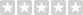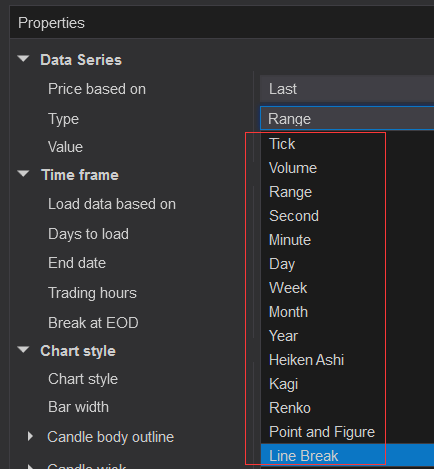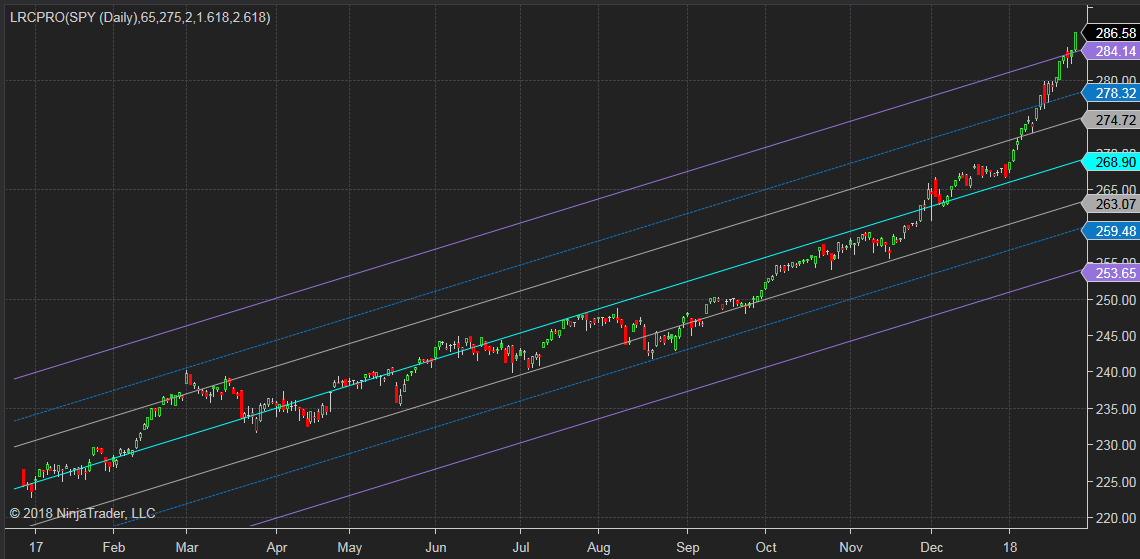Popular tags: Support, Resistance, Trend, Range, Fibonacci, Wave, Divergence, Multi time frames(MTF), Swing, Volume, Overbought, Oversold

Featured

# Linear Regression Channel indicator Pro version for NinjaTrader 8

Availability: In Stock
Price: \$99.00USD \$60.00USD
Qty:     - OR -(0/5)  0 reviews  |  Write a review

Please note that this purchase is a 1 Year license. License renew price will be 50% off for the 2nd year and after.

In statistics, linear regression is a linear approach for modelling the relationship between a scalar dependent variable y and one or more explanatory variables denoted X. The case of one explanatory variable is called simple linear regression. Wikipedia

Linear Regression Channel (LRC) is a very useful tool in trend analysis, especially for long term trend.

This Linear Regression Channel Pro version (LRC Pro) indicator has the following features:

• Adjust the number of bars (Period input) used in the calculation of Linear Regression Channel.
• Adjust the width of three channels with different values.
• Adjust the number of bars to extend to the right side, 0 means no extension.

It works on the following bar types:If you used Linear Regression Channel indicator before, you must know that the trendlines are not fixed. That trend is determined by calculating a Linear Regression Trendline using the least squares method.

With different 'Period' input value, the Linear Regression Trendlines vary greatly, let see an example of how LRC changes over time.

The chart below is taken from 1/26 with 'Period' =275, the Close price is above the 3rd upper trendline.The chart below is taken from 2/5 with 'Period' =150, the Close price on 1/26 is still above the 3rd upper trendline, but the three Highs before 1/26 are within the trendline now.

The Low on 2/5 is below the 3rd lower trendline.The chart below is taken from 2/5 with 'Period' =275, the Close price on 1/26 is still above the 3rd upper trendline, The Low on 2/5 is above the 3rd lower trendline.

The images below are random screenshots.

You can also use more than one instance with different inputs.

## Write a review

Your Review: Note: HTML is not translated!

Enter the code in the box below:Latest
Welcome to Patternsmart
Converting your indicator from one platform to another.